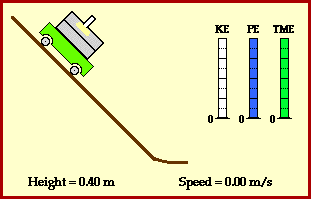## Energy Conservation on an Incline

Consider an ordinary lab cart loaded with bricks and accelerating down an inclined plane. How could work and energy be utilized to analyze the motion of the loaded cart? Would the total mechanical energy of the cart be altered in the process of rolling down the incline? Or would the total mechanical energy of the cart merely be conserved?

Of course the answers to these questions begin by determining whether or not there are any external forces doing work upon the loaded cart. If external forces do work upon the cart, the total mechanical energy of the cart is not conserved; the initial amount of mechanical energy is not the same as the final amount of mechanical energy. On the other hand, if external forces do not do work upon the loaded cart, then the total mechanical energy is conserved; that is, mechanical energy is merely transformed from the form of potential energy to the form of kinetic energy while the total amount of the two forms remains unchanged.

In the case of the cart rolling down the incline, there are three external forces (the normal force, the force of friction and air resistance) and one internal force (the force of gravity). The normal force does not do work upon the cart because it acts in a direction perpendicular to the direction of motion. In such instances, the angle between F and d is 90 degrees and the work done by the force is 0 Joules. The force of friction does not do work upon the cart because it acts upon the wheels of the cart and actually does not serve to displace either the cart nor the wheels. The friction force only serves to help the wheels turn as the cart rolls down the hill. Friction only does work upon a skidding wheel. Finally, the force of air resistance does do work upon the cart; air resistance does negative work upon the cart since it acts in a direction opposite the direction of the cart's motion. Sometimes referred to as a dissipative force, air resistance contributes to a loss in the total amount of mechanical energy possessed by the cart. Subsequently, it would be expected that there would be a small amount of energy loss as the cart rolls down the hill from an elevated position to a position just above the ground.

Due to the difficulty in measuring air resistance forces and due to the small amount of existing Fair in situations in which a streamlined object moves at relatively low speeds, the affect of air resistance is often neglected. If air resistance is neglected, then it would be expected that the total mechanical energy of the cart would be conserved. The animation below depicts this phenomenon (in the absence of air resistance).As the cart rolls down the hill from its elevated position, its mechanical energy is transformed from potential energy to kinetic energy. At a height just above the ground, the majority of the energy is in the form of kinetic energy. This is to say, energy due to vertical position has been transformed into energy due to motion. In fact, if air resistance can be neglected, then the amount of potential energy loss equals the amount of kinetic energy gained. Calculations can be performed to illustrate that the potential energy lost by the 3.0-kg cart in the above animation is equal to the kinetic energy gained. Assume that the height of the cart changes from 0.40 meters to 0.05 meters and the speed changes from 0 m/s to 2.62 m/s.

For more information on physical descriptions of motion, visit The Physics Classroom Tutorial. Detailed information is available there on the following topics:

Potential Energy

Kinetic Energy

Mechanical Energy

Internal vs. External Forces

Work

Work-Energy Theorem

Work-Energy Bar Charts